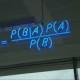# What’s next: seq2seq models

The short blog contains my notes from Seq2seq Tutorial. Please leave comments if you are interested in this topic.

Seq2seq model is a typical model which takes sequences as inputs and another sequence as the outputs. What are sequences? Think about an article as a sequence of words or a video file as a sequence of images. The model has been successfully applied in many NLP tasks like machine translation, summarization, etc. In terms of machine translation, we take a sequence of English words, for example, and we wish to translate the sentence into French, thus the output would be a sequence of French words. For summarization, we can take a long article as the input sequence and the output sequence would be the summary of the article, say the headline of a news.

### Language Models

To grasp the idea of seq2seq model, we should know the two prerequisite topics: word embeddings and recurrent language models. Word embeddings transform a single word into a dense vector (my old post to review) so we can have the inputs as a sequence of vectors. Now we will take a look at the recurrent language models.

There was an early work A Neural Probabilistic Language Model talking about neural language models. In general, as the tutorial shows:

If we want to predict the target word (“mat”) in this case, we should know all previous word sequence, so then the conditional probability gives the distribution of the target words and we will need the one with the maximum probability. Also, it is shown here:where$w_i^j$ stands for the subsequence of$w_i$ to$w_j$. For a short sequence which contains only 3 words, we can simply write it as:$\hat P (w_1,w_2,w_3) = \hat P(w_1) \hat P(w_2|w_1)\hat P(w_3|w_1,w_2)$

With n-gram models, the current word is only related to its previous (n-1) words. By applying the chain rule, we have:where you can imagine in our previous case,$\hat P(w_3|w_1,w_2)\approx\hat P(w_3|w_2)$.

Typically, a seq2seq model consists of an encoder and a decoder. In the literature of deep learning, we use RNNs for both components. The following screenshot shows a nice example:The blue part shows the encoder which takes a sequence (ABCD) as inputs, and$v$ is the hidden state at the last timestamp. The purple part shows the decoder who outputs a sequence (XYZQ) by taking the last hidden state of encoder v as its initial hidden state. So here v is considered to have all the information of the input sequence.

To formulate the model,$x_1..x_T$ is our input sequence, the output or target sequence is$y_1..y_{T'}$, because we would have different lengths ($T ,T'$) for the sequences. The goal is to find the optimal target sequence which maximizes the conditional probability.

### The vanilla encoder and decoder

Following nice graphs are from here.

Keep in mind that there is a softmax operation in the decoder part before the outputs since it is generating a probability distribution over the vocabulary.

### Drawbacks

• Generate word by word from left to right. It is natural that we are generating the sequence word by word at each timestep, however, we may miss the best sequence (combination of the words). We can apply beam search to tackle this problem. For more info please check this paper: Beam Search Strategies for Neural Machine Translation
• Fixed size embedding. The vector$v$ captures all the information of the input sequence. Is it too much? There is a better way: Attention Mechanism!

Some tutorials on seq2seq models with code:
Tensorflow NMT
PyTorch Translation with attention (I do recommend this!)

In the following posts,  I will introduce:

Keep calm and update blog.

## 3 thoughts on “What’s next: seq2seq models”

1.jnscollier says:

I am VERY interested in learning all I can about sequence models. I just haven’t dedicated the time in-depth to actually understand it deeply! How are you learning this? Are you academically educated or is this self-learning? Your posts are high quality.

Like

1.Irene says:

Hi, thanks for leaving me the comment! I had some related background (say math, ML, etc) then I could start reading the papers directly or, go to some blog posts lol Wish you good luck 🙂

Like